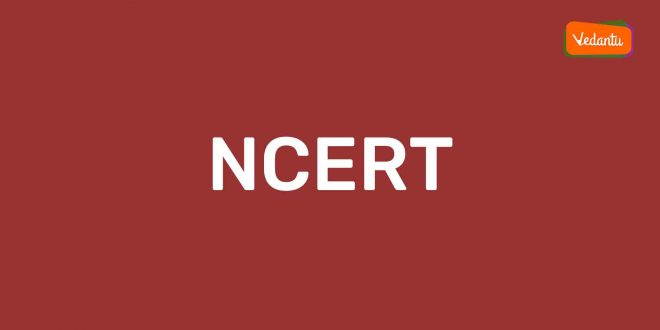Friday , July 30 2021# Notable Solution from Chapters 1-4 for Maths NCERT Class 10

The 10th standard for any student is considered to be one of the primary leaps before one gets ready for bigger hurdles in the future. Since it is the first major board examination for all the candidates studying in class 10, there would be a few butterflies in your stomach. To ease your never we are here to help you out with some key solutions.

For a few individuals, Mathematics is one particular subject which acts as an Achilles heel, but if you are confident enough you will surely be on the winning side. To make things transparent for you, vedantu’s NCERT solution for class 10 Maths is sure to bring hay days for you. We will discuss in detail that will help you to approach the first four chapters of your syllabi in a seamless manner.

Our solution ideally helps you to understand the chapters in a simple manner. We help you to get a grasp of all the key chapters in a nutshell and we will analyse the pivotal arise from where questions are bound to come. There is a high possibility that all the areas are not going to be touched by the majority of the candidates.

• We have assimilated some of the imperative areas that can help you go ahead with clear thinking.
• As per the pattern of examination out of 100 marks 80 marks are to be attended for theoretical applications and 20 marks for internal assessment.
• To score quantum marks in the theoretical section, focus mainly on objective questions.
• The total number of objective questions has been raised to 30 from 40 without altering the final or total marks.
• Here you can find across the list of the first four chapters included in the class-10 maths syllabus with a proper insight into all the topics covered under them.
1. Real Numbers- Chapter-1

The commencement chapter of the maths syllabus in class-10 is an extension of all the topics that you have learnt in class-9. Remember section 1.2 & 1.3 in this chapter contain key characteristics of positive integers. Here you will come across Euclid’s division algorithm along with the fundamental theorem of arithmetic.

In section 1.4 you will need to study irrational numbers yet again followed by decimal expansion which is laid in the following section. The section relating to Euclid’s division of algorithm has a lot to offer with divisibility of integers. Numerous applications are completely associated with divisibility characteristics of integers. This first chapter is ideal and advantageous to compute HCF relating to a couple of integers.

1. Polynomials- Chapter-2

There are four exercises in total from this particular section; however, the final exercise is optional for you.  In the very first exercise, you will have to find zeros of polynomials. In the next exercise, you can rest assured that a couple of questions will be asked.

You need to confirm the relationship that exists between zeros and coefficients in the first instance. In the second instance, you need to find across the quadratic polynomial. In the third exercise, there are a total of five questions and you need to carry out a division of polynomials and ascertain zeros of polynomials. In the part containing optimal exercise, there would be five questions and here you need to find zeros of polynomials.

1. Pair of linear equation in a couple of variables – Chapter-3

There would be a total of seven exercises and the final chapter being optional. It is mostly the continuation of class 9. Some key topics covered in these areas are graphical representation along with a pair of a linear equation.

You will come across algebraic methods for solving a pair of a linear equation. Elimination and substitution methods are to be approached. Knowing about cross multiplication and equations reduced to a pair of linear equations in a couple of variables.